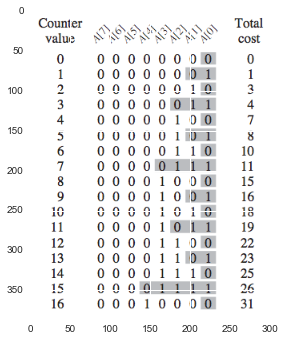In :
%matplotlib inline
import pandas as pd

import numpy as np
from __future__ import division
import itertools

import matplotlib.pyplot as plt
import seaborn as sns

import logging
logger = logging.getLogger()


# 17 Amortized Analysis¶

In an amortized analysis, we averget the time required to perform a sequence of data-structure operations over all the operations performed.

Amortized analsis differs from average-case analysis in that probability is not involved; an amortized analysis guarantees the _average performance of each operation in the worst case__.

Bear in mind that the charges assigned during an amortized analysis are for analysis purposes only.

When we perform an amortized analysis, we often gain insight into a particular data structure, and this insight can help us optimize the design.

### 17.1 Aggregate analysis¶

We show that for all $n$, a sequence of $n$ operations takes worst-case time $T(n)$ in total.

##### Stack operations¶

PUSH $geq$ POP + MULTIPOP
$1 \times O(n) = O(n)$

##### Incrementing a binary counter¶
In :
plt.imshow(plt.imread('./res/fig17_2.png'))

Out:
<matplotlib.image.AxesImage at 0x10941d850>In general, for $i = 0, 1, \dotsc, k-1$, bit $A[i]$ flips $\lfloor \frac{n}{2^i} \rfloor$ times in a sequence of $n$ INCREMENT operations on an initially zero counter.

\begin{align} \sum_{i=0}^{k-1} \lfloor \frac{n}{2^i} \rfloor &< n \sum_{i=0}^{\infty} \frac{1}{2^i} \\ &= 2n \end{align}

### 17.2 The accounting method¶

credit: the cost that an operation's amortized cost $\hat{c_i}$ exceeds its actual cost $c_i$.

requriments: $$\sum_{i=1}^{n} \hat{c_i} \geq \sum_{i=1}^{n} c_i$$

##### Stack operations¶
$c_i$ $\hat{c_i}$
PUSH 1 2
POP 1 0
MULTIPOP min(k,s) 0

$2 \times O(n) = O(n)$

##### Incrementing a binary counter¶

set a bit to 1: 2
set a bit to 0: 0

The INCREMENT procedure sets at most one bit, $2 \times O(n) = O(n)$

### 17.3 The potential method¶

Let $D_i$ be the data structure that results after applying the $i$th operation to data structure $D_{i-1}$.

potential function $\phi$: maps each data structure $D_i$ to a real number $\phi(D_i)$.

$\hat{c_i} = c_i + \phi(D_i) - \phi(D_{i-1})$
hence, the total amortized cost of the $n$ operations is: $$\sum_{i=1}^n \hat{c_i} = \sum_{i=1}^n c_i + \phi(D_n) - \phi(D_0)$$

Different potential functions may yield different amortized costs yet still be upper bounds on the actual costs. The best potential function to use depends on the disired time bounds.

##### Stack operations¶

define: $\phi$ to be the number of objects in the stack.

for PUSH: \begin{align} \hat{c_i} &= c_i + \phi(D_i) - \phi(D_{i-1}) \\ &= 1 + (s+1) - s \\ &= 2 \end{align}

for POP: \begin{align} \hat{c_i} &= c_i + \phi(D_i) - \phi(D_{i-1}) \\ &= 1 + (s-1) - s \\ &= 0 \end{align}

for MULTIPOP: \begin{align} \hat{c_i} &= c_i + \phi(D_i) - \phi(D_{i-1}) \\ &= k + (s-k) - s \\ &= 0 \end{align}

##### Incrementing a binary counter¶

define: $\phi$ to be $b_i$, the number of 1s in the counter after the $i$th operation.

Suppose: the $i$th INCREMENT operation reset $t_i$ bits.

for INCREMENT: \begin{align} \hat{c_i} &= c_i + \phi(D_i) - \phi(D_{i-1}) \\ &= (t_i + 1) + (b_{i-1} - t_i + 1) - b_{i-1} \\ &= 2 \end{align}

### 17.4 Dynamic tables¶

load factor: $$\alpha(T) = \frac{\|\text{items of T}\|}{\|T\|}$$

#### 17.4.1 Table expansion¶

insert an item into a full table, we expand the table with twice spaces.

The cost of the $i$th operation is: \begin{equation} c_i = \begin{cases} i \quad & \text{expand: if i - 1 is an exact power of 2} \\ 1 \quad & \text{otherwise} \end{cases} \end{equation}

The total cost of $n$ TABLE-INSERT operations is therefore: \begin{align} \sum_{i=1}^{n} c_i &\leq n + \sum_{j=0}^{\lfloor \lg n \rfloor} 2^j \\ &< n + 2n \\ &= 3n \end{align}

#### 17.4.2 Table expansion and contraction¶

Halve the table size when deleting an item causes the table to become less than 1/4 full, rather than 1/2 full as before(引起振荡).